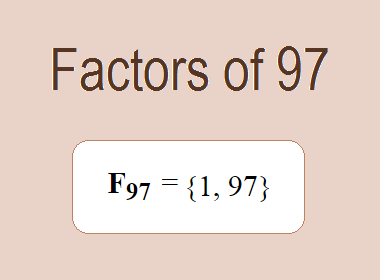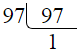# Factors of 97The factors of 97 are 1 and 97 i.e. F97 = {1, 97}. The factors of 97 are all the numbers that can divide 97 without leaving a remainder. 97 is a prime number, so it is divisible by 1 and 97 only.

We can check if these numbers are factors of 97 by dividing 97 by each of them. If the result is a whole number, then the number is a factor of 97. Let's do this for each of the numbers listed above:

·        1 is a factor of 97 because 97 divided by 1 is 97.

·        97 is a factor of 97 because 97 divided by 97 is 1.

## Properties of the Factors of 97

The factors of 97 have some interesting properties. One of the properties is that the sum of the factors of 97 is equal to 98. We can see this by adding all the factors of 97 together:

1 + 97 = 98

Another property of the factors of 97 is that the only prime factor of 97 is 97 itself.

********************

********************

## Applications of the Factors of 97

The factors of 97 have several applications in mathematics. One of the applications is in finding the highest common factor (HCF) of two or more numbers. The HCF is the largest factor that two or more numbers have in common. For example, to find the HCF of 97 and 194, we need to find the factors of both numbers and identify the largest factor they have in common. The factors of 97 are 1, and 97. The factors of 194 are 1, 2, 97, and 194. The largest factor that they have in common is 97. Therefore, the HCF of 97 and 194 is 97.

Another application of the factors of 97 is in prime factorization. Prime factorization is the process of expressing a number as the product of its prime factors. The prime factor of 97 is 97 since it is only the prime number that can divide 97 without leaving a remainder. Therefore, we can express 97 as:

97 = 97

We can do prime factorization by division method as given below,97 = 97

Since 97 is a prime number, there is no factor tree of 97.

## Conclusion

The factors of 97 are the numbers that can divide 97 without leaving a remainder. The factors of 97 are 1, and 97. The factors of 97 have some interesting properties, such as having a sum of 98. The factors of 97 have several applications in mathematics, such as finding the highest common factor and prime factorization.